# KR100943617B1 - Estimating apparatus of harmonic state and estimation method for harmonic state - Google Patents

Estimating apparatus of harmonic state and estimation method for harmonic state Download PDF

## Info

Publication number
KR100943617B1
KR100943617B1 KR1020070097986A KR20070097986A KR100943617B1 KR 100943617 B1 KR100943617 B1 KR 100943617B1 KR 1020070097986 A KR1020070097986 A KR 1020070097986A KR 20070097986 A KR20070097986 A KR 20070097986A KR 100943617 B1 KR100943617 B1 KR 100943617B1
Authority
KR
South Korea
Prior art keywords
harmonic
state
calculating
harmonics
unit
Prior art date
Application number
KR1020070097986A
Other languages
Korean (ko)
Other versions
KR20090032615A (en
Inventor
곽노홍
명근식
박상호
박희철
왕용필
전영수
황갑철
Original Assignee
한국전력공사
Priority date (The priority date is an assumption and is not a legal conclusion. Google has not performed a legal analysis and makes no representation as to the accuracy of the date listed.)
Filing date
Publication date
Application filed by 한국전력공사 filed Critical 한국전력공사
Priority to KR1020070097986A priority Critical patent/KR100943617B1/en
Publication of KR20090032615A publication Critical patent/KR20090032615A/en
Application granted granted Critical
Publication of KR100943617B1 publication Critical patent/KR100943617B1/en

## Images

•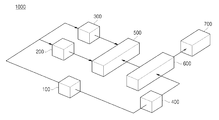•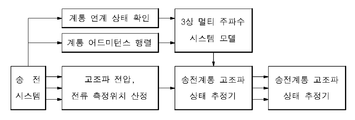•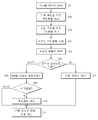•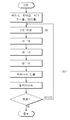•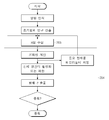•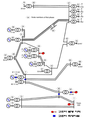•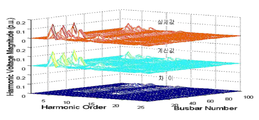•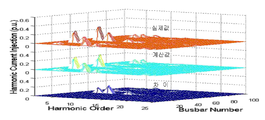## Classifications

• GPHYSICS
• G01MEASURING; TESTING
• G01RMEASURING ELECTRIC VARIABLES; MEASURING MAGNETIC VARIABLES
• G01R23/00Arrangements for measuring frequencies; Arrangements for analysing frequency spectra
• G01R23/16Spectrum analysis; Fourier analysis
• G01R23/20Measurement of non-linear distortion, e.g. harmonics or noise
• GPHYSICS
• G01MEASURING; TESTING
• G01RMEASURING ELECTRIC VARIABLES; MEASURING MAGNETIC VARIABLES
• G01R19/00Arrangements for measuring currents or voltages or for indicating presence or sign thereof
• G01R19/25Arrangements for measuring currents or voltages or for indicating presence or sign thereof using digital measurement techniques
• G01R19/2513Arrangements for monitoring electric power systems, e.g. power lines or loads; Logging
• HELECTRICITY
• H02GENERATION; CONVERSION OR DISTRIBUTION OF ELECTRIC POWER
• H02JCIRCUIT ARRANGEMENTS OR SYSTEMS FOR SUPPLYING OR DISTRIBUTING ELECTRIC POWER; SYSTEMS FOR STORING ELECTRIC ENERGY
• H02J3/00Circuit arrangements for ac mains or ac distribution networks
• H02J3/01Arrangements for reducing harmonics or ripples
• H02J2203/20

## Abstract

The present invention provides a harmonic state estimator and an harmonic state estimating method with increased accuracy and reliability in order to effectively cope with harmonic disturbance problems. Harmonic estimating apparatus according to the present invention is a linkage status check unit for checking the system linkage state with the data measured in the transmission system, admittance calculation unit for calculating the admittance matrix value with the data measured in the power transmission system, data measured in the transmission system Position calculation unit for calculating the measurement position of the harmonic, multi-frequency calculation unit for calculating the fundamental frequency and the frequency for each order from the data received from the linkage status check unit and the admittance calculation unit and the position calculation unit and the multi-frequency calculation A first harmonic state estimator for estimating the state of harmonics from an output value from a negative portion, and an output unit for outputting a harmonic voltage value and a harmonic current value from the state of harmonics estimated by the first harmonic state estimator.
Harmonics, Harmonic Disorders, Harmonic Estimation, Error Calculation, Immune Algorithm, Genetic Algorithm

## Description

Harmonic state estimation device and harmonic state estimation method {ESTIMATING APPARATUS OF HARMONIC STATE AND ESTIMATION METHOD FOR HARMONIC STATE}

The present invention relates to a harmonic state estimating apparatus and a harmonic state estimating method, and more particularly, to a harmonic state estimating apparatus and a harmonic state estimating method having increased accuracy and reliability in order to effectively cope with a harmonic disturbance problem.

Designing a measurement system to perform harmonic state estimation of a power system is a very complex problem. Since the measuring system is composed of a large number of measuring devices and a complicated communication system, the mixing of various noises in the measuring process or the communication process can be a problem, and on the other hand, the system is observed due to the failure of the measuring equipment or the communication system. It may become impossible or bad condition to reach an unreliable situation. As a countermeasure, a large number of measuring devices can be installed to collect a large amount of measurement data. However, increasing the number of measuring devices in the measuring system increases the accuracy and reliability of the condition estimation, while increasing the investment cost of the measuring equipment. Will increase.

In addition, the conventional transmission system harmonic state estimation method is to select the measurement position using the network topology (Network Topology). Network Topology is a method of selecting an optimal measurement position by repeating trial and error. It takes a very long time to select a measurement position, and an error occurs when estimating a state.

Since the Korean power transmission system is a complex power system, if the harmonic state estimation is performed by this trial and error method, a calculation error may occur if the optimal position is not selected, and thus the reliability of the estimated value is somewhat lowered.

The present invention provides a method of estimating the state of harmonics by selecting an installation position of the measurement equipment so as to minimize the error of the harmonic state estimation result using a minimum measurement equipment.

The present invention also provides a method for implementing an intelligent harmonic state estimation algorithm and developing an intelligent state estimation system to enable accurate state estimation for harmonics of a transmission system.

Harmonic estimating apparatus according to the present invention, the linkage status check unit for checking the system linkage status with the data measured in the transmission system, the admittance calculation unit for calculating the admittance matrix value with the data measured in the transmission system, measured in the transmission system Position calculation unit for calculating the measurement position of the harmonics as data, Multi-frequency calculation unit for calculating the fundamental frequency and the frequency for each order from the data received from the linkage status check unit and the admittance calculation unit and the position calculation unit and the multi-frequency A first harmonic state estimator for estimating the state of harmonics from an output value from the calculator, and an output unit for outputting harmonic voltage values and harmonic current values from the harmonic states estimated by the first harmonic state estimator.

In this case, the harmonic state estimator includes an input unit for receiving data, an additional unit for adding a measurement matrix for measuring current to the input data, and adding a measurement matrix for voltage measurement, and an actual measurement voltage matrix from an output value from the adder. A transform unit for selecting and converting the transform into an actual value matrix, a first control unit determining whether harmonic state estimation is possible from the actual value matrix, and an equation using singular value decomposition when the first control unit determines that the harmonic state estimation is impossible. The second control unit may be configured to determine whether a measurement error is generated by calculating a and an error calculator configured to calculate a measurement error when the second control unit detects a measurement error.

In addition, when the first controller determines that the harmonic state can be estimated, the harmonic voltage and the harmonic current may be measured by calculating a general equation.

In addition, the harmonic estimating apparatus may further include a second harmonic state estimator for applying the estimated harmonic state to an immune algorithm or a genetic algorithm.

On the other hand, the harmonic state estimation method according to the present invention, calculating the system link state and the admittance matrix value from the data measured in the transmission system, and calculating the measurement position of the harmonic voltage and harmonic current from the data measured in the transmission system Estimating the state of harmonics from the admittance matrix value and the output value of the measurement position information, and finally calculating the harmonic voltage and harmonic current from the estimated state of harmonics.

At this time, in the step of estimating the state of the harmonics, when it is not possible to estimate the state of the harmonics, an equation using singular value decomposition may be calculated and a measurement error may be calculated when an error occurs. In addition, after the step of estimating the state of the harmonics may further comprise the step of further applying an immune algorithm or genetic algorithm.

The present invention can estimate the state of harmonics by selecting the installation position of the measurement equipment so as to minimize the error of the harmonic state estimation result using the minimum measurement equipment.

In addition, since the optimum measurement position selection algorithm is used, reliable calculation results can be obtained when estimating harmonic states.

In addition, by implementing an intelligent harmonic state estimation algorithm and developing an intelligent state estimation system, it is possible to accurately estimate the harmonics of the transmission system.

Hereinafter, exemplary embodiments of the present invention will be described in detail with reference to the accompanying drawings so that those skilled in the art may easily implement the present invention. As can be easily understood by those skilled in the art, the embodiments described below may be modified in various forms without departing from the concept and scope of the present invention. All terms including technical terms and scientific terms used hereinafter have the same meanings as commonly understood by one of ordinary skill in the art. Terms defined in advance are further interpreted to have a meaning consistent with the related technical literature and the presently disclosed contents, and are not interpreted in an ideal or very formal sense unless defined. In order to clearly describe the present invention, parts irrelevant to the description are omitted.

Hereinafter, a harmonic state estimating apparatus and a harmonic state estimating method according to an embodiment of the present invention will be described.

1 is a view schematically showing a harmonic state estimating apparatus according to an embodiment of the present invention.

As shown in FIG. 1, the harmonic state estimating apparatus 1000 according to an embodiment of the present invention includes a linkage state checking unit 200 and a transmission line system 100 confirming a linkage state of the power transmission system 100. From the admittance calculation unit 300 for calculating the admittance matrix value from the data measured at the position calculation unit 400 for preliminarily calculating the measurement position of harmonics, the linkage status check unit 200 and the admittance calculation unit 300. First harmonic state estimator 600 and harmonics for estimating the state of the harmonics from the output values of the multi-frequency calculator 500, the multi-frequency calculator 500, and the position calculator 400 for calculating a frequency from the received data. And an output unit 700 for outputting a voltage value and a harmonic current value.

2 is a flowchart illustrating a harmonic state estimation method according to an embodiment of the present invention. As shown in FIG. 2, the linkage state checking unit 200 and the admittance calculating unit 300 check the grid connection state with the voltage and current measured by the power transmission system 100, and check the grid admittance. Find the matrix value. Based on this value, the harmonic voltage and current measurement positions are selected in the three-phase multi-frequency region including the fundamental frequency and the system for each order. Subsequently, the output harmonic state is finally estimated using the first harmonic state estimator 600 using the output values of the position calculating unit 400 and the multi-frequency calculating unit 500.

3 is a flowchart illustrating the function of the first harmonic state estimation unit 600 according to an embodiment of the present invention.

As shown in FIG. 3, the first harmonic state estimation unit 600 according to an embodiment of the present invention includes an input step 201 for receiving data and a measurement matrix for measuring current on the input data. In addition, additional steps 202 and 203 for adding a measurement matrix for voltage measurement, and conversion steps 204 and 205 for selecting an actual measurement voltage matrix from an output value from the additional step and converting the actual measured voltage matrix into an actual value matrix.

In addition, the first harmonic state estimator 600 according to an embodiment of the present invention, the first control step 206, the first control step 206 to determine whether the harmonic state estimation is possible from the actual value matrix In the case that it is determined that the harmonic state estimation is impossible, the second control step 209 of calculating an equation using singular value decomposition (SVD) (208) and determining whether a measurement error has occurred is performed. If a measurement error is found in step 209, an error calculation step 210 of calculating a measurement error is included. In this case, when it is determined that the harmonic state estimation is possible in the first control step 206, the general equation is calculated (207) to calculate the harmonic voltage and the harmonic current (211).

The basic algorithm of harmonic state estimation is shown by reference numerals 201 to 205. Conventional algorithms are divided into algorithms for measuring the entire system and for measuring a portion of the system, but the improved harmonic estimating apparatus according to an embodiment of the present invention is a method of correcting an error occurring when a portion is measured. Was added (210). Therefore, as shown in FIG. 2, the harmonic state can be estimated under any condition as in the case of measuring the entire system or measuring a part of the system.

4 is a flowchart illustrating a genetic algorithm to which a harmonic state estimation method is applied according to another embodiment of the present invention, and FIG. 5 is a flowchart illustrating an immune algorithm to which a harmonic state estimation method is applied according to another embodiment of the present invention.

As shown in Figs. 4 and 5, the harmonic state estimator included in the harmonic state estimation apparatus according to another embodiment of the present invention inherits the state of harmonics estimated by the first harmonic state estimator 600 described above. Apply to algorithm 302 and immunoalgorithm 304. As a result, an intelligent harmonic estimation algorithm can be derived that is more optimized for measuring the harmonic position.

6 is a diagram showing the application of an intelligent harmonic state estimation apparatus to a transmission system.

The rectangular point is the optimal measurement point selected by the intelligent state estimation algorithm, and the harmonic generation region indicated by the arrow can be found through the state estimation. In other words, it is possible to find the harmonic generation region by selecting the optimum measuring point like the rectangular point without measuring all the regions, and to estimate the harmonic state in the places where the measuring equipment is not installed.

FIG. 7 is a graph showing the harmonic voltage state estimation result according to the optimum measurement position selection, and FIG. 8 is a graph showing the harmonic current state estimation result according to the optimum measurement position selection. As shown, the harmonic estimating apparatus according to the present invention can confirm the effect that the deviation from the actual value hardly occurs.

In the above, it is confirmed that the accuracy and reliability of the harmonic state estimation of the transmission system can be improved while maintaining the economics in terms of the installation cost of the instrument.

As described above, the present invention enables the selection of an optimal measuring point for accurate estimation of harmonic states of a transmission system, thereby minimizing the number of installations of instruments, and by establishing an appropriate harmonic countermeasure at each point through accurate harmonic state estimation, Can significantly reduce economic losses.

What has been described above is just one embodiment for implementing the harmonic estimating apparatus and the harmonic estimating method according to the present invention, and the present invention is not limited to the above-described embodiment, as claimed in the following claims. Without departing from the gist of the invention, those skilled in the art belong to the technical idea of the present invention to the extent that various changes can be carried out.

1 is a view schematically showing a harmonic state estimating apparatus according to an embodiment of the present invention.

2 is a flowchart illustrating a harmonic state estimation method according to an embodiment of the present invention.

3 is a flowchart illustrating the function of the first harmonic state estimation unit 600 according to an embodiment of the present invention.

4 is a flowchart illustrating a genetic algorithm to which a harmonic state estimation method is applied according to another embodiment of the present invention.

5 is a flowchart illustrating an immune algorithm to which a harmonic state estimation method is applied according to another embodiment of the present invention.

6 is a diagram showing the application of an intelligent harmonic state estimation apparatus to a transmission system.

7 is a graph showing the harmonic voltage state estimation result according to the selection of the optimum measurement position.

8 is a graph illustrating a harmonic current state estimation result according to selection of an optimum measurement position.

## Claims (7)

1. Linkage status checker to check the system linkage status with the data measured on the transmission system,
An admittance calculation unit for calculating an admittance matrix value from the data measured in the power transmission system;
Position calculation unit for calculating the measurement position of harmonics using the data measured in the transmission system,
A multi-frequency calculation unit for calculating a fundamental frequency and a frequency for each order from data received from the linkage state checking unit and the admittance calculating unit;
A first harmonic state estimator for estimating a state of harmonics from the position calculation unit and the output values from the multi-frequency calculating unit, and
An output unit for outputting a harmonic voltage value and a harmonic current value from a state of harmonics estimated by the first harmonic state estimation unit,
The first harmonic state estimating unit,
Input unit for receiving data,
An adder for adding a measurement matrix for voltage measurement by adding a measurement matrix for current measurement to the input data;
A converter which selects a real measured voltage matrix from an output value from the adder and converts the actual measured voltage matrix into an actual value matrix;
A first controller for determining whether harmonic state estimation is possible from the actual value matrix;
A second controller which determines whether a measurement error occurs by calculating an equation using singular value decomposition when the first controller determines that the harmonic state estimation is impossible;
And an error calculator for calculating a measurement error when the second control unit detects a measurement error.
2. delete
3. The method of claim 1,
And a harmonic state estimating apparatus for calculating harmonic voltages and harmonic currents by calculating a general equation when the first controller determines that the harmonic state can be estimated.
4. The method of claim 1,
And a second harmonic state estimator for applying the estimated harmonic state to an immune algorithm or a genetic algorithm.
5. Calculating a system linkage state and an admittance matrix value using the data measured in the transmission system, and calculating a measurement position of harmonic voltage and harmonic current using the data measured in the transmission system,
Estimating a state of harmonics from the admittance matrix value and the output value of the measurement position information, and
Finally calculating a harmonic voltage and a harmonic current from the estimated harmonic state,
The harmonic state estimation method of calculating the equation using singular value decomposition when the state of the harmonics is not possible in estimating the state of the harmonics, and the measurement error when an error occurs.
6. delete
7. The method of claim 5,
And applying the estimated state of harmonics to an immune algorithm or a genetic algorithm after estimating the state of harmonics.
KR1020070097986A 2007-09-28 2007-09-28 Estimating apparatus of harmonic state and estimation method for harmonic state KR100943617B1 (en)

## Priority Applications (1)

Application Number Priority Date Filing Date Title
KR1020070097986A KR100943617B1 (en) 2007-09-28 2007-09-28 Estimating apparatus of harmonic state and estimation method for harmonic state

## Applications Claiming Priority (1)

Application Number Priority Date Filing Date Title
KR1020070097986A KR100943617B1 (en) 2007-09-28 2007-09-28 Estimating apparatus of harmonic state and estimation method for harmonic state

## Publications (2)

Publication Number Publication Date
KR20090032615A KR20090032615A (en) 2009-04-01
KR100943617B1 true KR100943617B1 (en) 2010-02-24

# Family

## Family Applications (1)

Application Number Title Priority Date Filing Date
KR1020070097986A KR100943617B1 (en) 2007-09-28 2007-09-28 Estimating apparatus of harmonic state and estimation method for harmonic state

## Country Status (1)

KR (1) KR100943617B1 (en)

## Families Citing this family (2)

* Cited by examiner, † Cited by third party
Publication number Priority date Publication date Assignee Title
CN103323688B (en) * 2012-03-20 2015-11-25 华北电力科学研究院有限责任公司 A kind of harmonic state estimation method and apparatus of electric system
KR102049899B1 (en) * 2013-09-06 2019-11-28 한국전력공사 Harmonic source estimation apparatus and method

## Citations (4)

* Cited by examiner, † Cited by third party
Publication number Priority date Publication date Assignee Title
KR20020068215A (en) * 2001-02-20 2002-08-27 학교법인 성균관대학 Method for detecting high impedance fault using wavelet transformation and for measuring fault distance using thereof
KR20030029036A (en) * 2001-10-04 2003-04-11 가부시키가이샤 히타치세이사쿠쇼 Measurement method of leakage current or resistance, and monitor apparatus thereof, and system thereof
JP2003244851A (en) 2002-02-20 2003-08-29 Nissin Electric Co Ltd Method of detecting single operation of distributed power source
JP2006204069A (en) * 2005-01-24 2006-08-03 Kansai Electric Power Co Inc:The Individual operation detecting method and individual operation detecting device

## Patent Citations (4)

* Cited by examiner, † Cited by third party
Publication number Priority date Publication date Assignee Title
KR20020068215A (en) * 2001-02-20 2002-08-27 학교법인 성균관대학 Method for detecting high impedance fault using wavelet transformation and for measuring fault distance using thereof
KR20030029036A (en) * 2001-10-04 2003-04-11 가부시키가이샤 히타치세이사쿠쇼 Measurement method of leakage current or resistance, and monitor apparatus thereof, and system thereof
JP2003244851A (en) 2002-02-20 2003-08-29 Nissin Electric Co Ltd Method of detecting single operation of distributed power source
JP2006204069A (en) * 2005-01-24 2006-08-03 Kansai Electric Power Co Inc:The Individual operation detecting method and individual operation detecting device

## Also Published As

Publication number Publication date
KR20090032615A (en) 2009-04-01

## Similar Documents

Publication Publication Date Title
Asiminoaei et al. A digital controlled PV-inverter with grid impedance estimation for ENS detection
Yao et al. Wide-area damping controller for power system interarea oscillations: A networked predictive control approach
Lotfifard et al. Voltage sag data utilization for distribution fault location
US4812995A (en) Adaptive Kalman Filtering in fault classification
US8081002B2 (en) Apparatus and method for determining location of phase-to-phase fault or three-phase fault
US20020072867A1 (en) System and method for locating a disturbance in a power system based upon disturbance power and energy
US20030040846A1 (en) Stability prediction for an electric power network
Alimardani et al. Distribution system state estimation based on nonsynchronized smart meters
US20130135907A1 (en) Harmonic current suppression method and harmonic current suppression device of power conversion device
Filomena et al. Fault location for underground distribution feeders: An extended impedance-based formulation with capacitive current compensation
FR2865038A1 (en) Device for measuring a synchronous vector
US8198904B2 (en) Synchrophasor measuring device and inter-bus-line phase angle difference measurement unit using the same
Vanbeylen et al. Blind maximum likelihood identification of Hammerstein systems
CN101344567B (en) Method for determining location of phase-to-earth fault
CN101320073B (en) Method for determining location of phase-to-earth fault
JP2012029305A5 (en)
US6104182A (en) Method of deriving a signal indicating an oscillation in an electric power supply system
US7818159B2 (en) Intelligent system and method for monitoring generator reactive power limit using machine model parameters
Larsson et al. An overview of important practical aspects of continuous-time ARMA system identification
US20060173640A1 (en) System and method for determining harmonic contributions from non-linear loads without disconnecting any load
Valdivia et al. Black-box modeling of three-phase voltage source inverters for system-level analysis
US8532230B2 (en) Instantaneous phasor determination for poly-phase electrical grids
JP6025575B2 (en) System and method for three-phase power flow analysis
Hayes et al. A closed-loop state estimation tool for MV network monitoring and operation
Panteli et al. Assessing the effect of failures in the information and communication infrastructure on power system reliability

## Legal Events

Date Code Title Description
A201 Request for examination
E902 Notification of reason for refusal
E701 Decision to grant or registration of patent right
GRNT Written decision to grant
FPAY Annual fee payment

Payment date: 20130201

Year of fee payment: 4

FPAY Annual fee payment

Payment date: 20140203

Year of fee payment: 5

FPAY Annual fee payment

Payment date: 20150202

Year of fee payment: 6

FPAY Annual fee payment

Payment date: 20160202

Year of fee payment: 7

FPAY Annual fee payment

Payment date: 20170201

Year of fee payment: 8

FPAY Annual fee payment

Payment date: 20180202

Year of fee payment: 9